• Version 8
Using Random Rule and Sampling Rule Steps
• 02 Nov 2023
• Dark
Light

# Using Random Rule and Sampling Rule Steps

• Dark
Light

Article Summary

## Overview

The Random Rule and Sampling Rule components provide a way to test for probability within a Flow. Both steps randomly return one of two outcomes as True or False. The outcomes can be weighted by configuring the percentage of times that the outcome will be True.

To guarantee the ratio of "True" to "False" outcomes, the Sampling Rule offers an additional option for configuring the sample size via the Sample Key Input.

## Key Differences

The key difference between using the Sample Rule and a Random Rule is that a Sample Rule will evenly distribute the results around the Sample Key value.
For example, if the Sample Key value is 10, the Rule will travel down each path exactly 5 times for every 10 runs.

## Random Rule

The following example will utilize a Random Rule and Show Popup step that displays the outcome path.

1. Create a new Flow. Navigate to Toolbox > Data > Rules.
2. Add the Random Rule step to the workspace and define the Percent True input as 50.
This will weigh the odds that the Rule will be evaluated as True.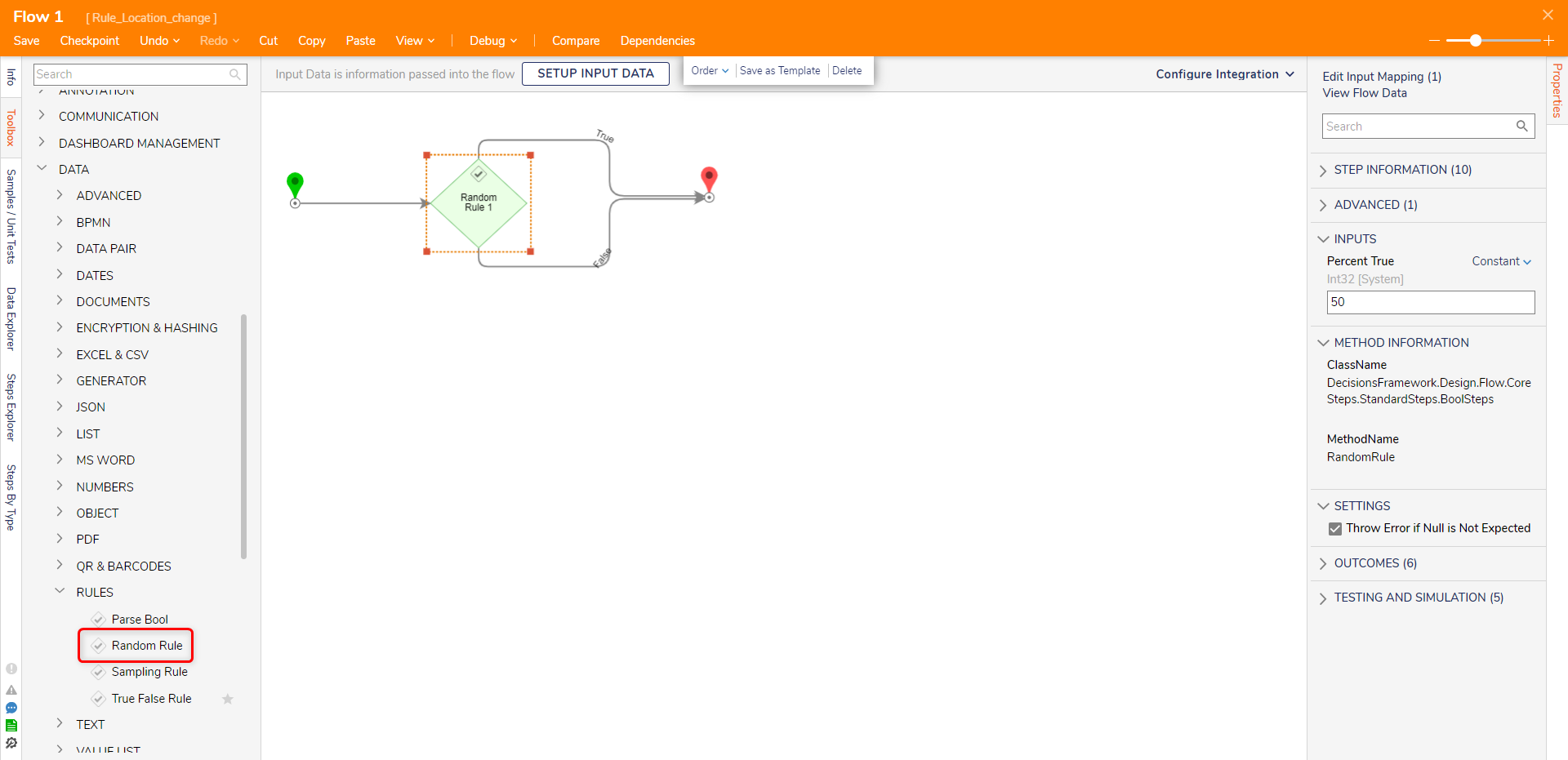3. Add the Show Popup step to both outcome paths and define the step.
For the Subject and Message inputs, input the popup step's path.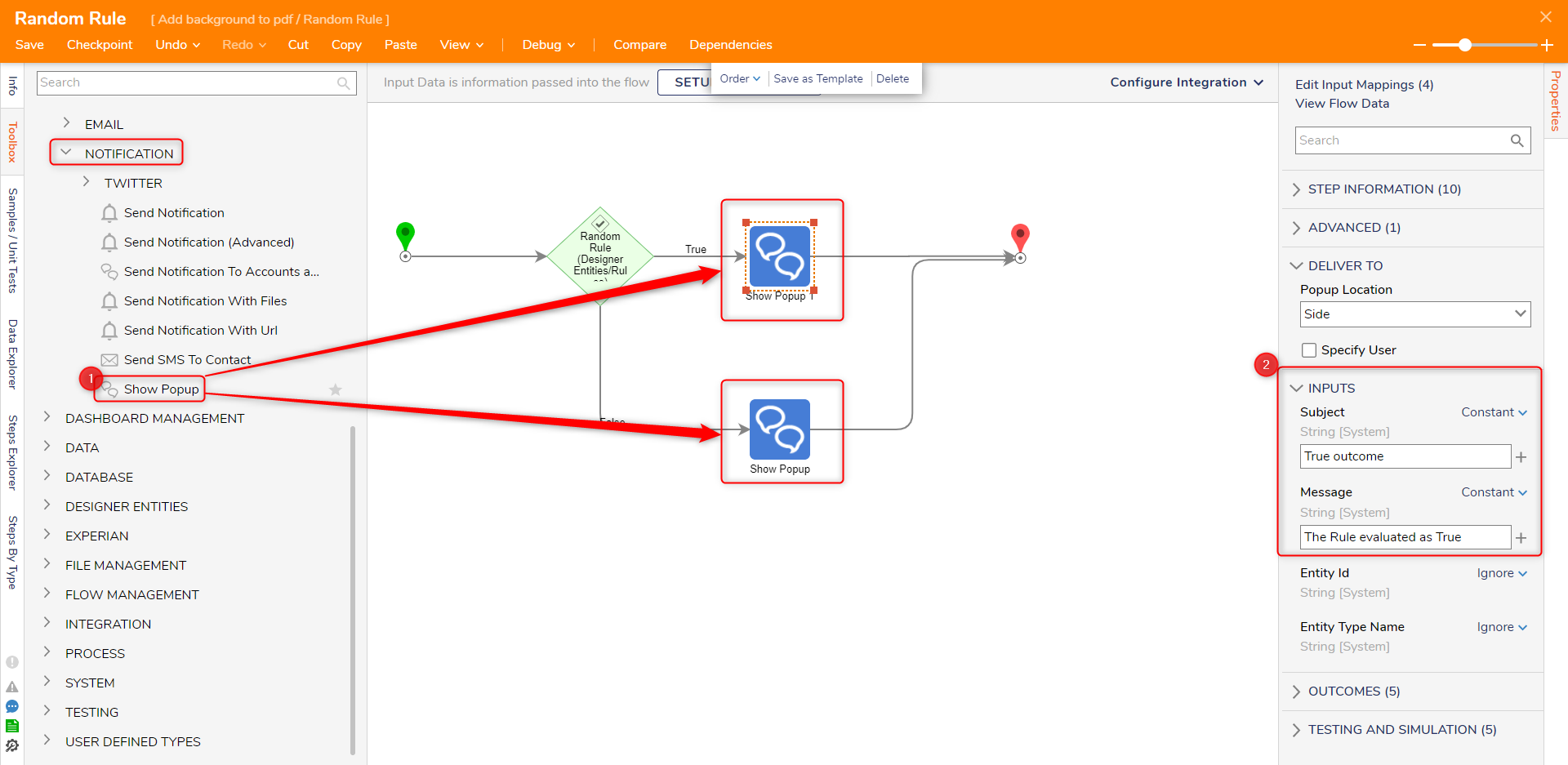4.  Debug the Flow. A notification will appear containing the result of the Rule.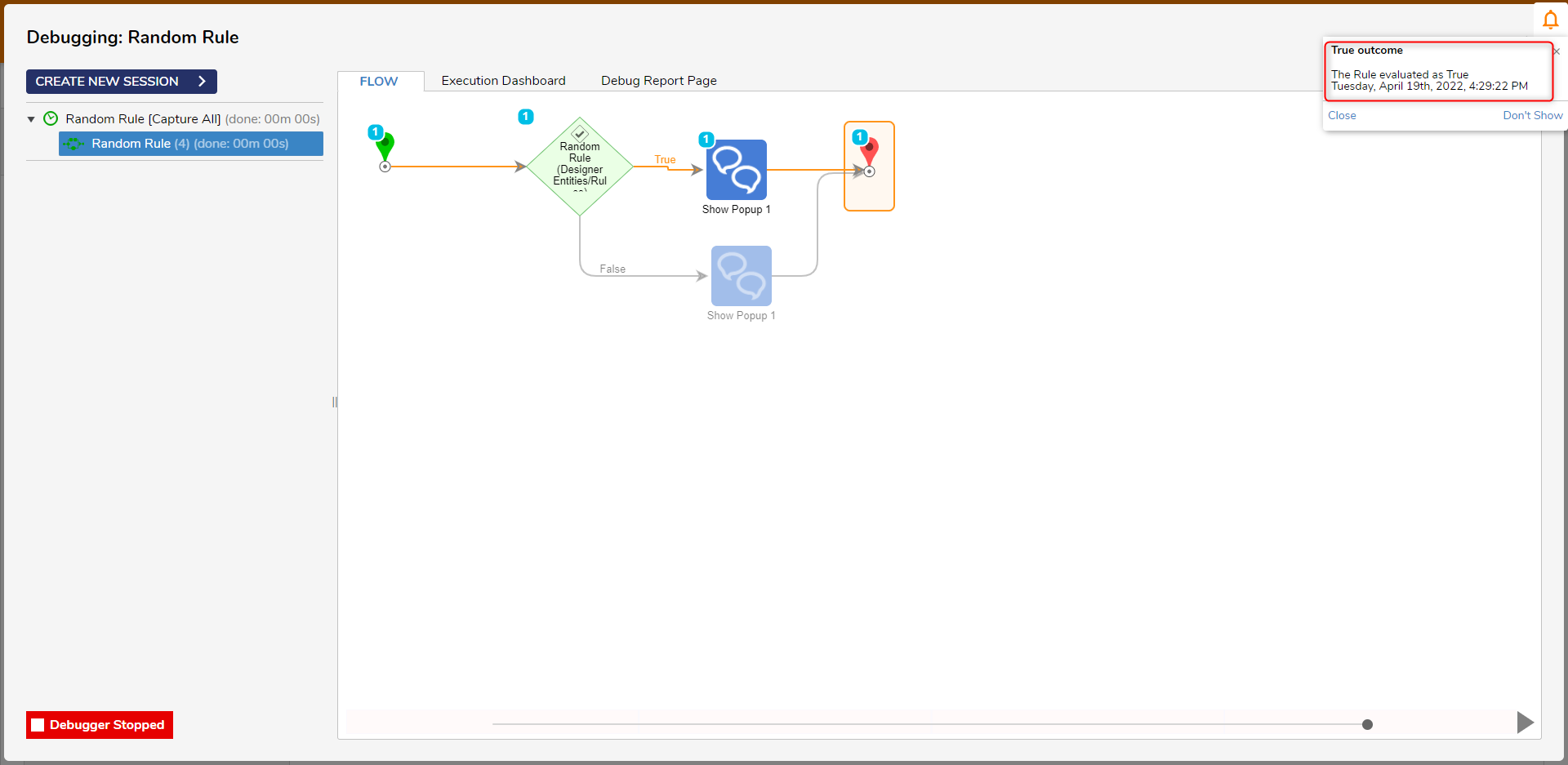## Sample Rule

1. With the Flow Designer > Toolbox > Data > Rules.
2. Add the Sampling Rule step to the workspace.
3. In the Sampling Rule step, define the Percent True as 50 and Sample Key as 10.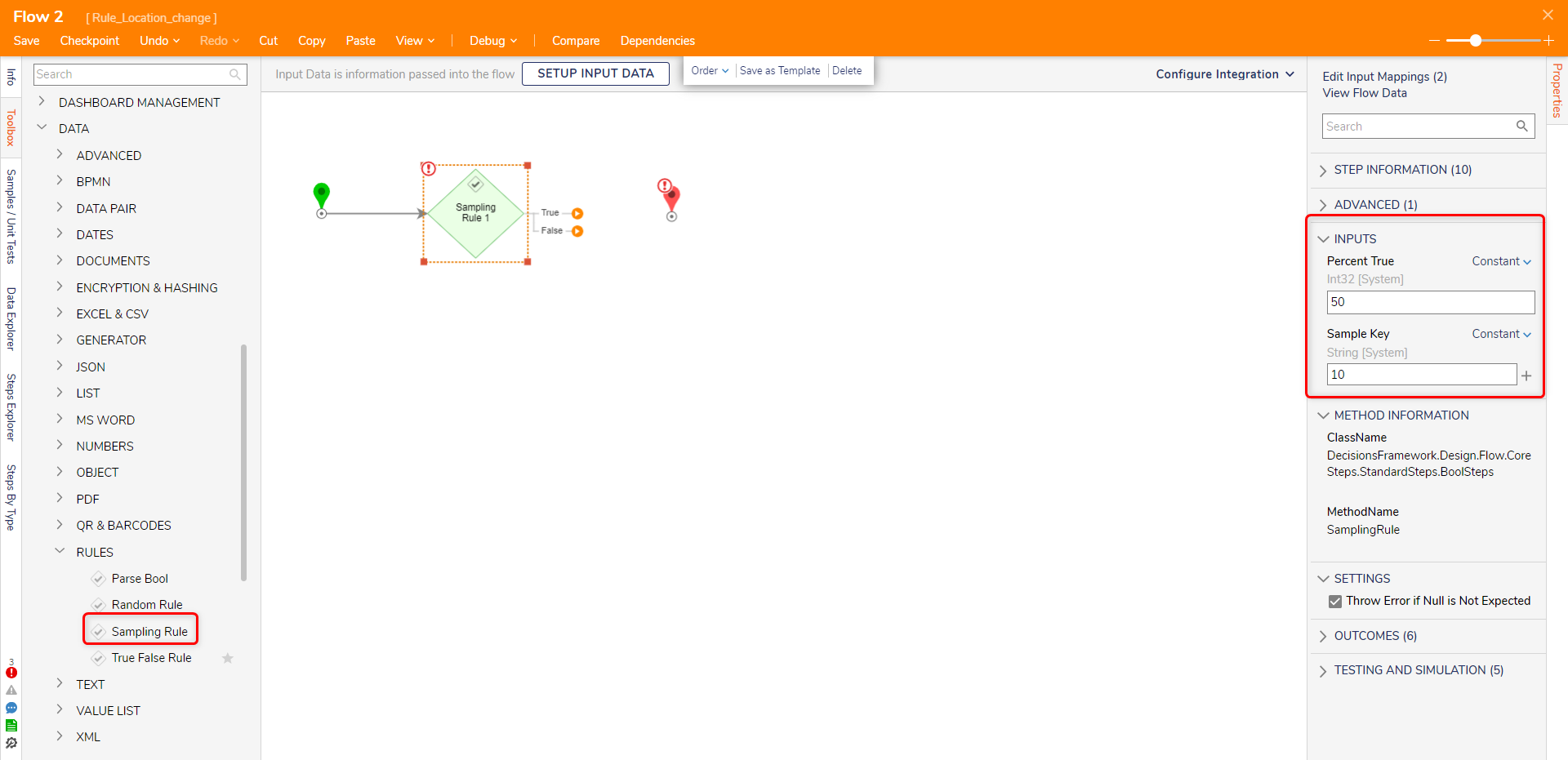4. In the Outcomes header for the step, check Output Outcome Name.
This defines a String labeled OutputPathName, which holds the name of the outcome path of the Flow.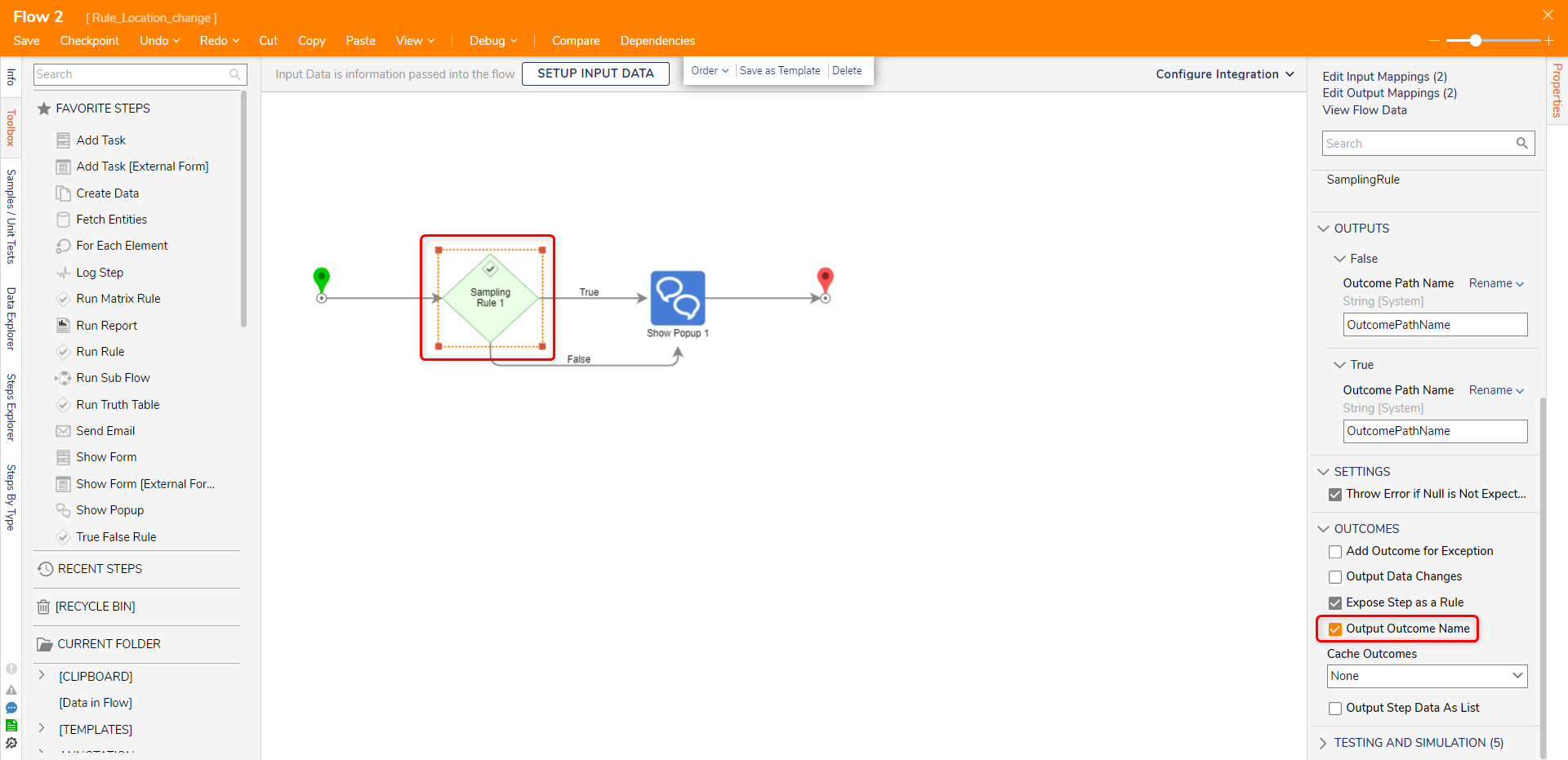5. Add the Show Popup step to both paths. Define the inputs to the Show Popup step. For the Subject, the Outcome Path is used.
For Message, use Select From Flow and choose OutcomePathName as its input.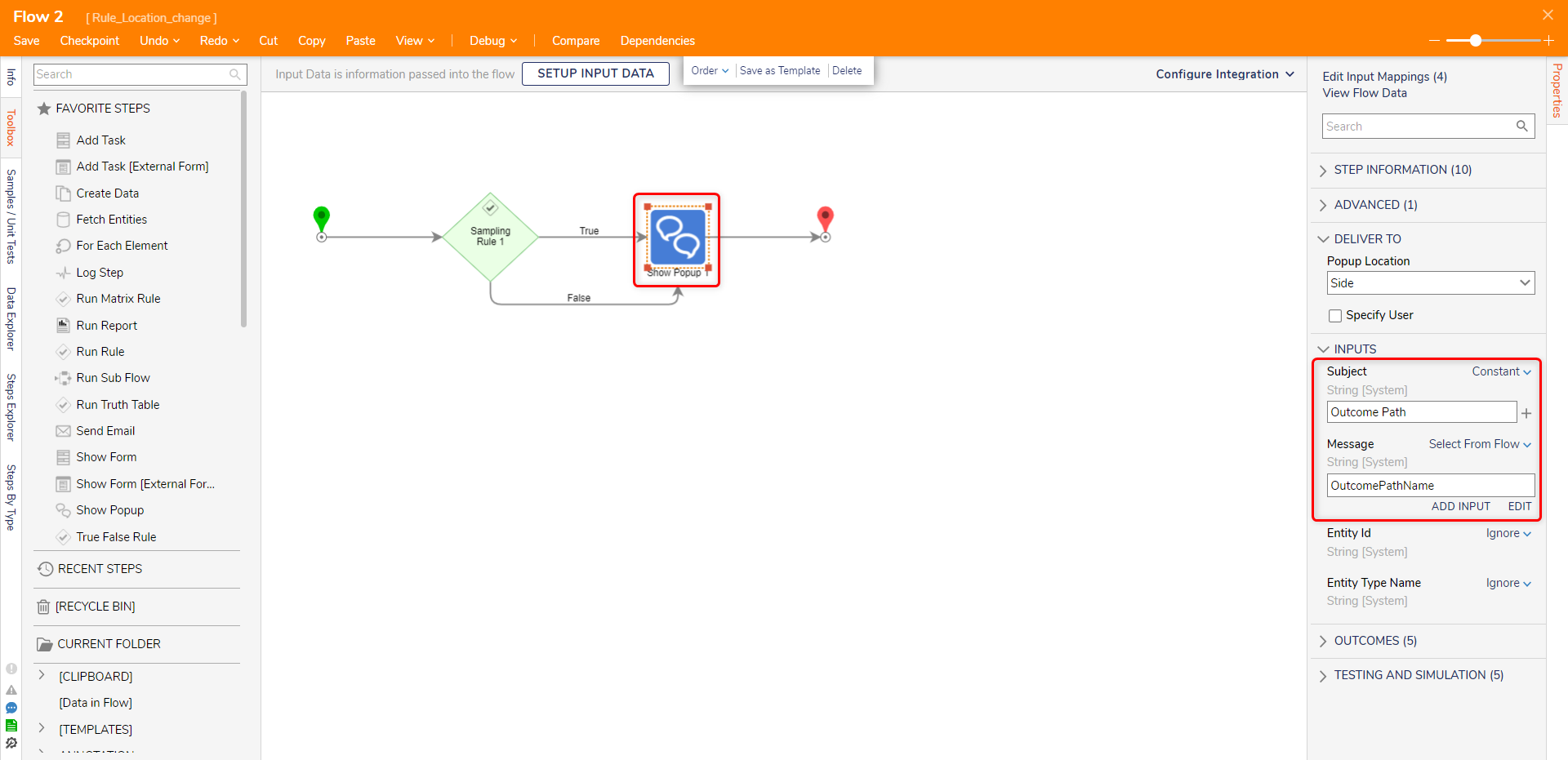6. Debug the Flow. A notification will appear highlighting the outcome path.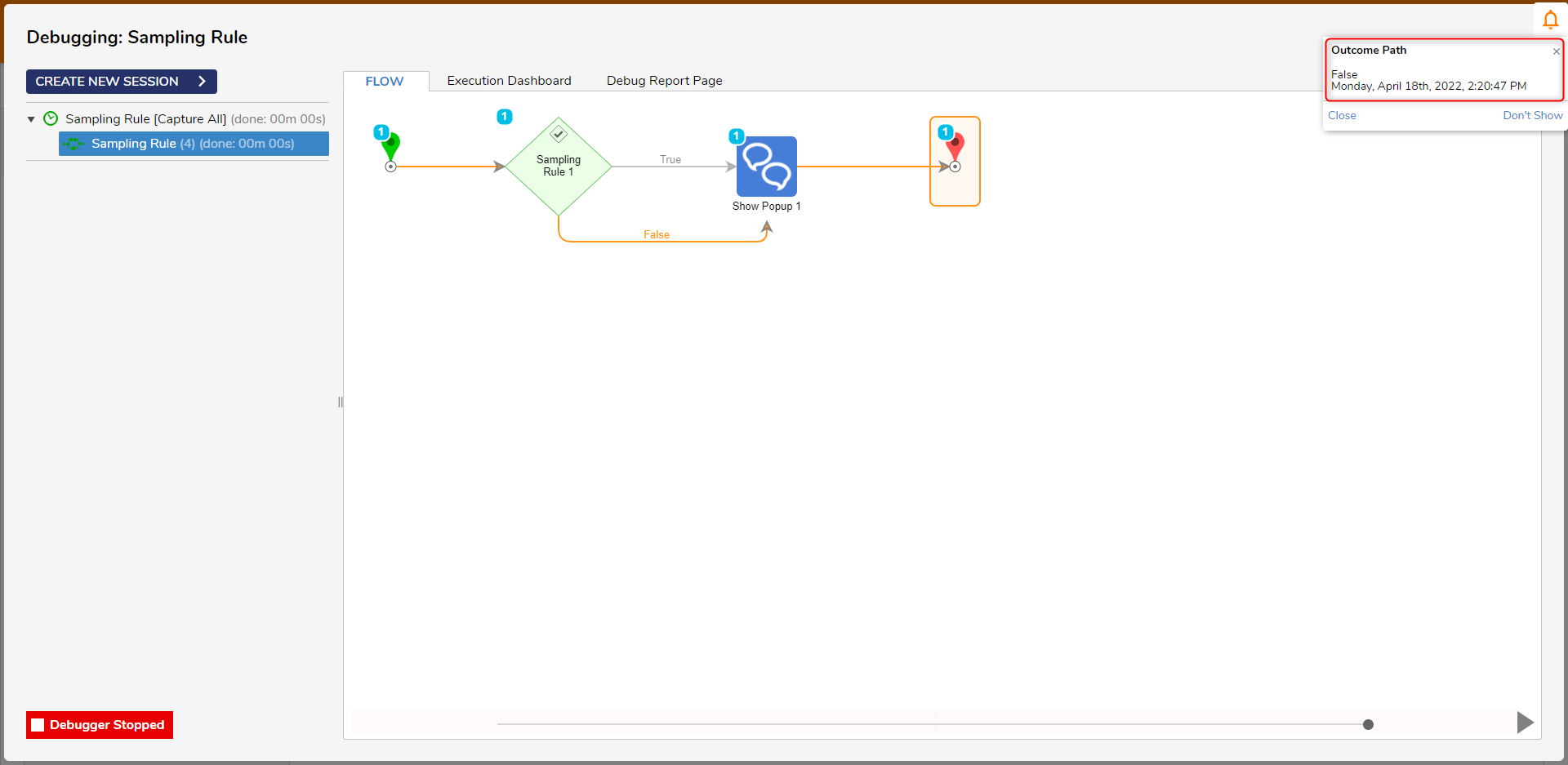For further information on Rules, visit the Decisions Forum.